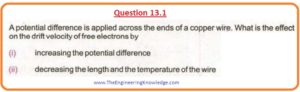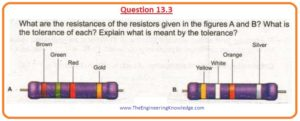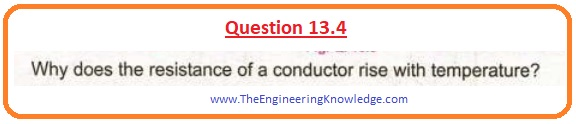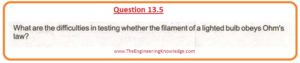Hello friends, I hope you all are doing great. In today’s tutorial, we will have a look at 2nd Year Physics Chapter 13 Solved Questions. I have started a series of tutorials related to solution of questions given in 2nd-year physics. In the previous tutorial, I have discussed all questions of chapter 12 with the detailed.

In this post, we will have a detailed look at all the questions given in chapter 13 of FSC physics. I will explain all questions one by one and give an answer to every question in a simple way. So let’s get started with 2nd Year Physics Chapter 13 Exercise Solved Questions.

#### 2nd Year Physics Chapter 13 Exercise Solved Questions

• So friends let’s discuss all the questions of chapter 13 one by one with the detailed.

#### Question 13.1• First of all, we discuss drift velocity it is a velocity that electrons needed in the existence of the electrical field provided by the input voltage source.

(i):

• With the increment in potential difference the drift velocity of electrons increases.

(ii):

• As we know that

R=pL/A

• From this equation, you can observe that with the decrement in length ‘L’ resistance decreases.
• With the decrement in temperature resistance also decreases.
• So resistance decrement increases the drift velocity of electrons.

#### Question 13.2• As we know this relation.

R=pL/A

• From this equation, you can see that resistance ‘R’ depend on ‘p’ length of the conductor, and area of that material.
• So any bend in the wire will not affect ‘p’, length and area of a wire. so there will be no effect on the resistance of material.

#### Question 13.3(A):

• If you look at first resistor that you will note that its first color is brown which means (1), second is green which means (5), for a third band (red), there are two zeros.
• The fourth band is gold whose tolerance is puls minus five percent.
• So resistance value for this first resistor will be 1500Ω+-5%.
• The resistance of this resistor is 1500Ω and +-5% is tolerance.

(B):

• For the second resistor first band is yellow which means 4, the second color is white which means ‘9’, the third is orange and there will be three zeros for this color.
• 4th is silver for which tolerance is +-10%.
• So the resistance of this resistor will be 49000Ω+-10%.
• The resistance of this resistor is 49000Ω and +-5% is tolerance.
• Tolerance describes how much changes in the value of given resistance can be done.
• For example, 49000Ω+-10% for any resistor resistance value is 49000Ω and in this value, +-10% variation can occur that called tolerance.

#### Question 13.4• The resistance offered by any conductive material is due to the collision of moving electrons with the vibrating atoms of the conductor.
• With the increment in temperature, the amplitude of vibrations of atoms increases creates difficulty for flow of electrons. In this way, resistance increases with temperature.

#### Question 13.5• Ohm’s law applicable for such conductive material whose resistance remains constant with the variation in voltage provided.

I∝V

• But the resistance of a lighted bulb does not remain constant bur increases with the increment in temperature.
• So filament of the bulb does not fulfill the Ohm’s law.

#### Question 13.6• As we know that.

P=V2/R

R=V2/P

• By submitting the given values in this equation.

R1=2202/500

=96.8Ω

R2=2202/100

=484Ω

• From the above results, you can see that R1 is less than R2. So we can conclude that resistance offered by the 500-watt bulb will be less than the 100-watt bulb.

#### Question 13.8• The relationship between the potential difference of battery and its emf is given here.

Vt=E- Ixr

• With the decrement in current the potential difference across the interior resistance of battery increases.
• As the emf of battery has constant so (Ixr) increment, it decreases Vt of battery. According to this equation (Vt=E- Ixr).

So friends that is a complete post about 2nd Year Physics Chapter 13 Exercise Solved Questions. I have solved all the questions given in the exercise of chapter 13 of physics. If you have any queries about this post ask in comments. Thanks for reading. So I will upload the solution of chapter 14 question.